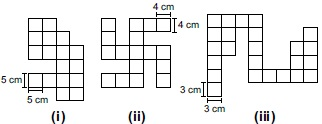# User ForumSubject :IMO    Class : Class 6

Find the perimeter of the following figures (not drawn to scale) and select the correct option.APerimeter of [(i) + (ii)] = Perimeter of [(ii) + (iii)]
BPerimeter of [(i) + (ii)] = Perimeter of (iii)
CPerimeter of [(i) + (iii)] > Perimeter of (ii)
DNone of these

What is the correct answer for this question and WHY?